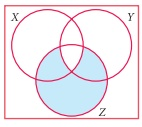Home | | Maths 9th std | Exercise 1.7: Multiple Choice Questions

# Exercise 1.7: Multiple Choice Questions

Maths : Set Language : Book Back, Exercise, Example Numerical Question with Answers, Solution : One Mark Choose the correct Answers : Exercise 1.7: Multiple Choice Questions

Exercise 1.7

Multiple Choice Questions

1. Which of the following is correct?

(1) {7} Ōłł {1,2,3,4,5,6,7,8,9,10}

(2) 7 Ōłł {1,2,3,4,5,6,7,8,9,10}

(3) 7 Ōłē {1,2,3,4,5,6,7,8,9,10}

(4) {7} ŌŖł {1,2,3,4,5,6,7,8,9,10}

2. The set P = {x | x Ōłł Ōäż , ŌĆō1< x < 1} is a

(1) Singleton set

(2) Power set

(3) Null set

(4) Subset

Solution: P = {0}

3. If U ={x | x Ōłł ŌäĢx < 10} and A = {x | x Ōłł ŌäĢ, 2 Ōēż x < 6} then (AŌĆ▓)ŌĆ▓ is

(1) {1, 6, 7, 8, 9}

(2) {1, 2, 3, 4}

(3) {2, 3, 4, 5}

(4) { }

Solution: (A') = A= {2, 3,4, 5}

4. If BŌŖå A then n(AŌł®B) is

(1) n(AŌĆōB)

(2) n(B)

(3) n(B ŌĆō A)

(4) n(A)

Solution: B ŌŖå A ŌćÆ AŌł®B = B

5. If A = {xyz} then the number of non- empty subsets of A is

(1) 8

(2) 5

(3) 6

(4) 7

Solution: Number of non-empty subsets =2-1=8-1=7

6. Which of the following is correct?

(1) Ōłģ ŌŖå {ab}

(2) Ōłģ Ōłł {ab}

(3) {a} Ōłł {ab}

(4) a ŌŖå {ab}

Solution: Empty set is an improper subset

7. If AŌł¬B = AŌł®B, then

(1) AŌēĀB

(2) A = B

(3) A ŌŖé B

(4)BŌŖéA

8. If B ŌĆō A is B, then AŌł®B is

(1) A

(2) B

(3) U

(4) Ōłģ

Solution: B - A = B ŌćÆ A and B are disjoint sets.

9. From the adjacent diagram n[P(AŌłåB)] is(1) 8

(2) 16

(3) 32

(4) 64

Solution: A ╬ö B = { 60, 85, 75, 90, 70}

ŌćÆ n(A ╬ö B) = 5

ŌćÆ n(P (A ╬ö B)) = 25 = 32

10. If n(A) = 10 and n(B) = 15, then the minimum and maximum number of elements in Ōł® B is

(1) 10,15

(2) 15,10

(3) 10,0

(4) 0,10

11. Let A = {Ōłģ} and B = P(A), then AŌł®B is

(1) {Ōłģ,{Ōłģ}}

(2) {Ōłģ}

(3) Ōłģ

(4) {0}

Solution: P(A) = { ├ś{├ś}}

[Answer: (2) { ├ś } ]

12. In a class of 50 boys, 35 boys play Carrom and 20 boys play Chess then the number of boys play both games is

(1) 5

(2) 30

(3) 15

(4) 10.

Solution: n(AŌł¬B) = n(A) + n(B) ŌĆö n(AŌł®B) ŌćÆ 50 = 35 + 20 ŌĆö n(AŌł®B) ŌćÆ n(AŌł®B) = 5

13. If U = {x : x Ōłł ŌäĢ and x < 10} , A = { 1,2,3,5,8 } and B = { 2,5,6,7,9 }, then n [(A Ōł¬ B)ŌĆ▓] is

(1) 1

(2) 2

(3) 4

(4) 8

Solution: U = {1, 2, 3,4, 5, 6, 7, 8, 9}

A = {1,2, 3, 5, 8}

B = {2, 5, 6, 7, 9}

AŌł¬B = {1,2, 3,5, 6, 7, 8, 9}

(AŌł¬B)' = {4},

n(AŌł¬B)' = 1

14. For any three sets P, Q and R, P ŌłÆ (Q Ōł® R) is

(1) P ŌłÆ(Q Ōł¬R)

(2) (P Ōł®Q)ŌłÆR

(3) (P ŌłÆQ)Ōł¬(P ŌłÆR)

(4) (P ŌłÆQ)Ōł®(P ŌłÆR)

Solution: P-(QŌł®R) = (P-Q)Ōł¬(P-R)

15. Which of the following is true?

(1) AŌłÆB =AŌł®B

(2) AŌłÆB=BŌłÆA

(3) (AŌł¬B)ŌĆ▓ =AŌĆ▓ Ōł¬BŌĆ▓

(4) (AŌł®B)ŌĆ▓ =AŌĆ▓ Ōł¬BŌĆ▓

Solution :

(1) (A-B) = AŌł®B   False

(2) A-B = B-A False

(3) (AŌł¬B)' = A' Ōł¬ B' False

(4) (AŌł®B)' = A' Ōł¬ B' True

[Answer: (4) (AŌł®B)' = A' Ōł¬ B']

16. If n (A Ōł¬ B Ōł¬C) = 100, n (A) = 4x, n (B) = 6x, n (C ) = 5x, n (A Ōł® B) = 20, n (B Ōł® C) = 15, n (A Ōł® C) = 25 and n(A Ōł® B Ōł®C) = 10 , then the value of x is

(1) 10

(2) 15

(3) 25

(4) 30

Solution:

n(AŌł¬BŌł¬C) = n(A) + n(B) + n(C) - n(AŌł®B) - n(BŌł®C) - n(CŌł®A) + n(AŌł®BŌł®C)

100 = 4x + 6x + 5x - 20 ŌĆō 15 -25 + 10

100 = 15x-60+10

100 = 15x-50

15x = 100 + 50 = 150

x = 10

17. For any three sets A, B and C, (A ŌłÆ B) Ōł® (B ŌłÆC) is equal to

(1) A only

(2) B only

(3) C only

(4) ŽĢ

Solution: (A - B) Ōł® (B - C) is equal to ŽĢ

18. If J = Set of three sided shapes, K = Set of shapes with two equal sides and L = Set of shapes with right angle, then J Ōł® K Ōł® L is

(1) Set of isoceles triangles

(2) Set of equilateral triangles

(3) Set of isoceles right triangles

(4) Set of right angled triangles

Solution:[Answer: (3) Set of isoceles right triangles]

19. The shaded region in the Venn diagram is(1) Z ŌłÆ(X Ōł¬Y)

(2) (X Ōł¬Y)Ōł®Z

(3) Z ŌłÆ(X Ōł®Y)

(4) Z Ōł¬(X Ōł®Y)

Solution: Z ŌĆō (X Ōł®Y)

[Answer: (3) Z ŌĆō (X Ōł®Y) ]

20. In a city, 40% people like only one fruit, 35% people like only two fruits, 20% people like all the three fruits. How many percentage of people do not like any one of the above three fruits?

(1) 5

(2) 8

(3) 10

(4) 15

Solution:40 + 35 + 20 + x = 100%

95% + x = 100%

x = 5%